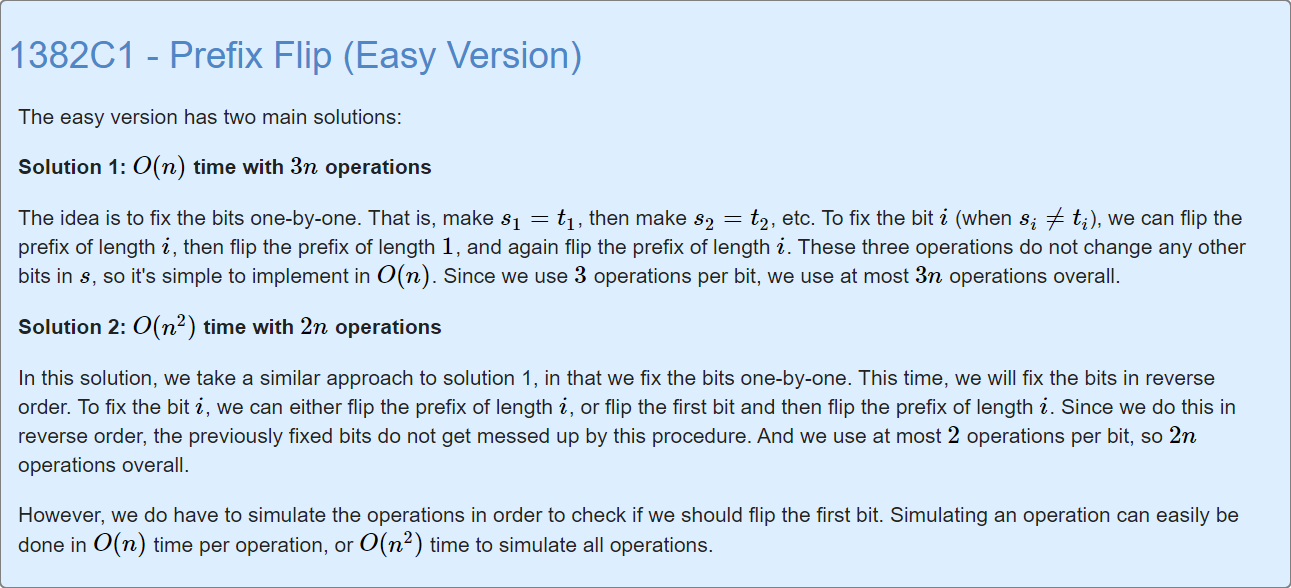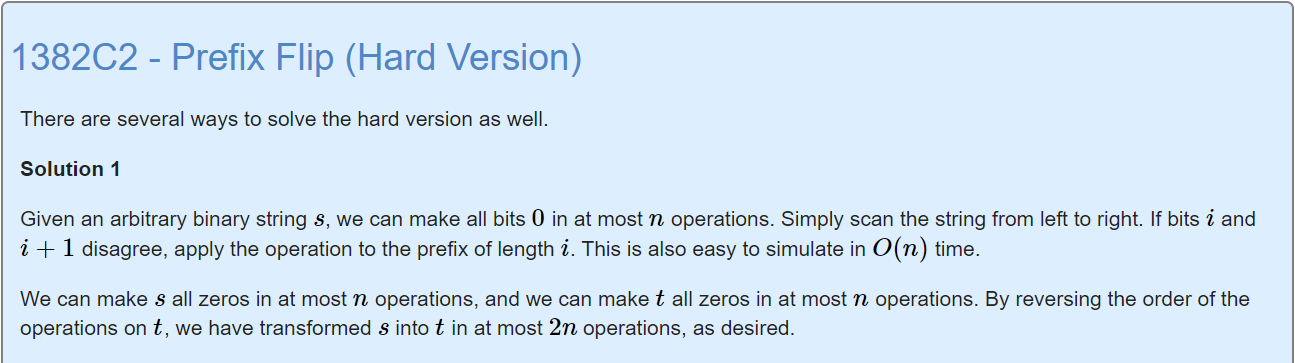## C1. Prefix Flip (Easy Version)

Easy Version，官方题解给出了两种做法。
Solution 1: O(n) time with 3n operations
Solution 2: O(n^2^) time with 2n operations// O(n^2), the number of operations are less than 2*n
#include <bits/stdc++.h>
using namespace std;
const int N=1e3+10;
int T,n;
char a[N],b[N];
vector<int>ans;
char f(char i) // 0->1, 1->0
{
return i=='1'?'0':'1';
}
void change(int l,int r) // O(n) per operation
{
int len=r-l+1;
int mid=(l+r)/2;
for(int i=l;i<=mid;i++)
{
int j=r+1-i;
if(a[i]==[j])
{
a[i]=f(a[i]);
a[j]=f(a[j]);
}
}
if(len&1)a[mid]=f(a[mid]);
}
int main()
{
ios::sync_with_stdio(false);
cin>>T;
while(T--)
{
cin>>n>>a+1>>b+1;
ans.clear();
for(int i=n;i>=1;i--)
{
if(a[i]!=b[i])
{
if(a[i]==a[j]) // 不用变a，只要变[1,i]
{
change(1,i);
ans.push_back(i);
}
else  // 变a之后，再变[1,i]
{
change(1,1);
change(1,i);
ans.push_back(1);
ans.push_back(i);
}
}
}
int sz=ans.size();
printf("%d",sz);
for(int i=0;i<sz;i++)
printf(" %d",ans[i]);
printf("\n");
}
return 0;
}


## C2. Prefix Flip (Hard Version)Hard Version也有几种做法，就介绍一下我认为最简单的做法吧。

// O(n), the number of operations are less than 2*n
#include <bits/stdc++.h>
using namespace std;
const int N=1e5+10;
int T,n;
char a[N],b[N];
vector<int>ans1,ans2;
void solve(char s[],vector<int> &g)
{
for(int i=1;i<=n-1;i++)
{
if(s[i]!=s[i+1])
g.push_back(i);
}
if(s[n]=='1')g.push_back(n); // 全1，再操作一次变成全0
}
int main()
{
ios::sync_with_stdio(false);
cin>>T;
while(T--)
{
cin>>n>>a+1>>b+1; // a,b下标从1开始
ans1.clear();
ans2.clear();
solve(a,ans1); // 注意传参是a，不是a+1
solve(b,ans2);
int sz1=ans1.size();
int sz2=ans2.size();
printf("%d",sz1+sz2);
for(int i=0;i<sz1;i++)
printf(" %d",ans1[i]);
for(int i=sz2-1;i>=0;i--) // 倒序输出
printf(" %d",ans2[i]);
printf("\n");
}
return 0;
}